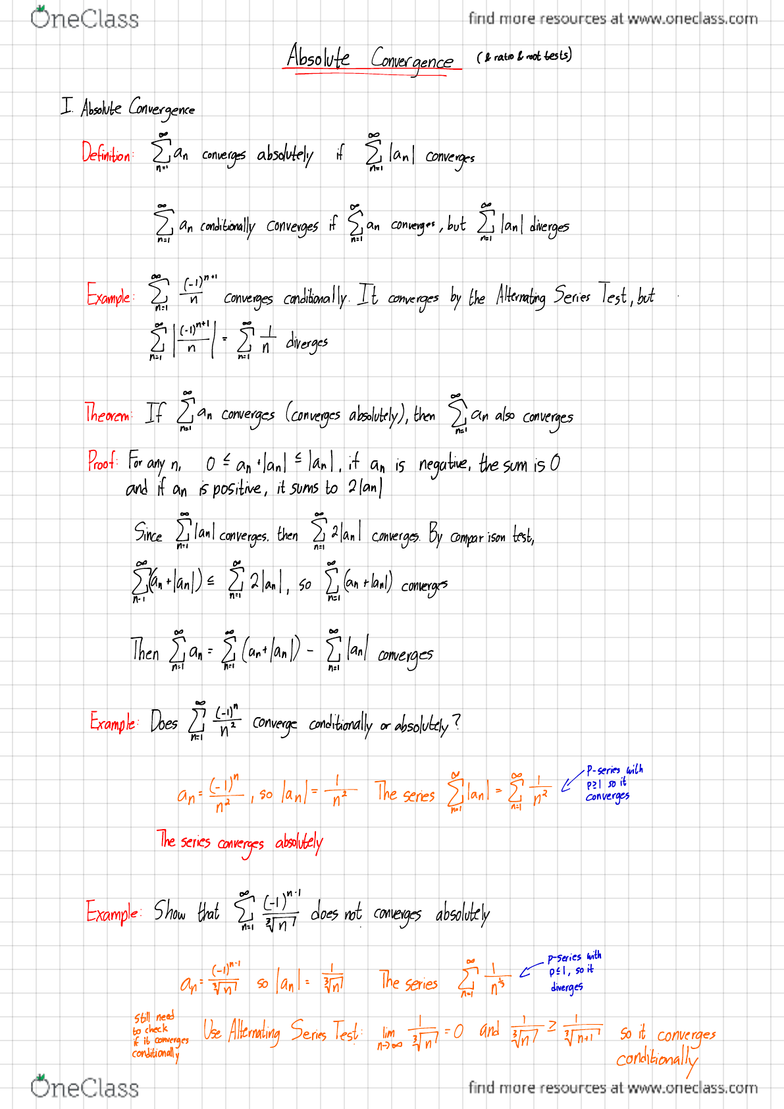Class Notes (1,100,000)
CA (620,000)
McMaster (50,000)
MATH (3,000)
Lecture 19

# Math1xx3 Lecture 19: Lecture 19

Department
Mathematics
Course Code
MATH 1XX3
Professor
Adam Van Tuyl
Lecture
19

Page:
of 3Absolutely (&ratio &root tests)
IAbsolute Convergence
Definition :an converges absolutely if {Ian Iconverges
an conditionally converges if ,
an converges ,but
,
Ian Idiverges
Example :,
¥""
converges conditionally .It converges by the Alternating
Series Test ,but .
If"÷t= Int diverges
Theorem :If §an
converges (converges absolutely),then {an also converges
Proof:For any n,an
ilanltlanl ,it an is negative ,the
sum is 0
and if an is positive ,it sums to 2Ian I
Since lanl converges .
then §Had
converges .By comparison lest,
dn
Han
De §Had ,so {Can
Hanl)converges
Then {an =§(ant
land -§lad converges
Example :Does §tj¥ converge conditionally or absolutely ?
anti,.lank 't The series sent qint HEIER
;
Is "
The series converges absolutely
Example :Show that Thin does not converges absolutely
ariftf so lank FA The series I'Ft¥d!EeH!t¥h
still need
tifotnhyexgqkwnegqes
Use Alternating
Series
Test :nhjmsstnn '
-0and ¥2 :# so it converges
conditionally
Series Ian
Diverge Converge
conditional absolute
Convergence convergence
:
IRatio Test
Definition :Given {an
!1!If
inns )Anf)=L >1 ,then Egan diverges
.
!2!If
him .
|Aan'÷I= Lcl ,then ,
an converges absolutely
!3!If him
,dAna÷/=l ,lest inclusive
Example :#
nest
msHtHnt÷÷pn=m.n¥ii¥¥=±m.Ymt¥=µo÷÷ .
Example :nfx
it
"(fn¥ an =fnt
ne
;mda÷tn%g¥=m±nn÷ .'s (4)
So it converges
.
#Root Test .
Definition :Given
,
an !
OFF him ,* tan =L >1
,series diverges
Off him
,.TH =L <1
,series converges absolutely
Off him ,* TH =L =1
,
series
inclusive .
Example :{ (¥÷)an=H¥o)
so him
.think .tk#n=him.HI= ÷u
So series converges
,
Example :Apply Ratio Test to ,'F
An,,
=ftp.np ,
hi
;sla÷lihn%¥t÷p=ni%cnn÷=i Inasive
To
know when this converges,we need to know the valve of P
.
Why does .this work ?
If him .FI/ =L ,this means for avery large N,
aa
.=L
:µ"
=an
L
ants =
@N+i
)L
=aµL2
ANT }t(
AN
+2 )L=aµL3
So for large N,series looks like XaµL+aµl2
+
aµL3 t
...
So for large N,the series looks like ageometric series .
50 convergence
,
depends upon L